# 老是自以为 JVM 懂了，那你知道 i = i++ 和 i = ++i 的区别吗？# 1、题目

package pers.mobian.questions01;public class test01 {    public static void main(String[] args) {        int i = 1;        i = i++;        int j = i++;        int k = i + ++i * i++;        System.out.println("i="+i);        System.out.println("j="+j);        System.out.println("k="+k);    }}

# 2、分析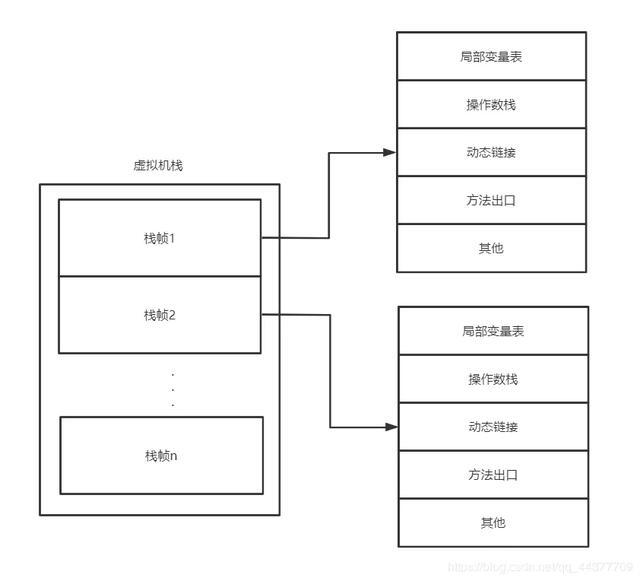# 2.1、第一步

int i = 1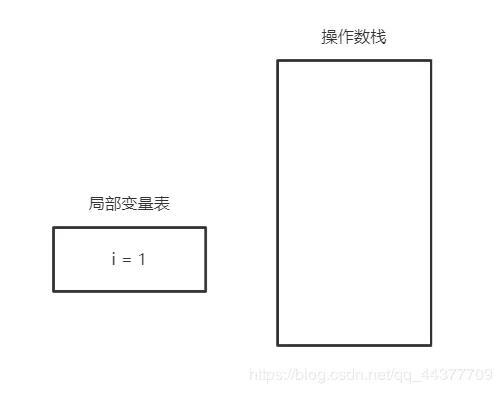# 2.2、第二步

i = i++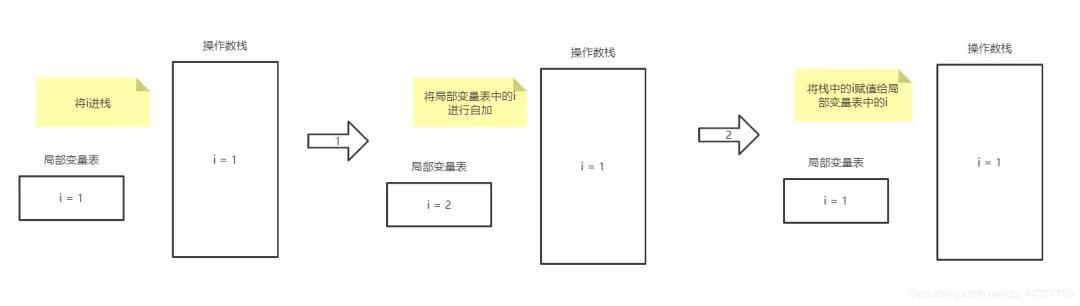# 2.3、第三步

int j = i++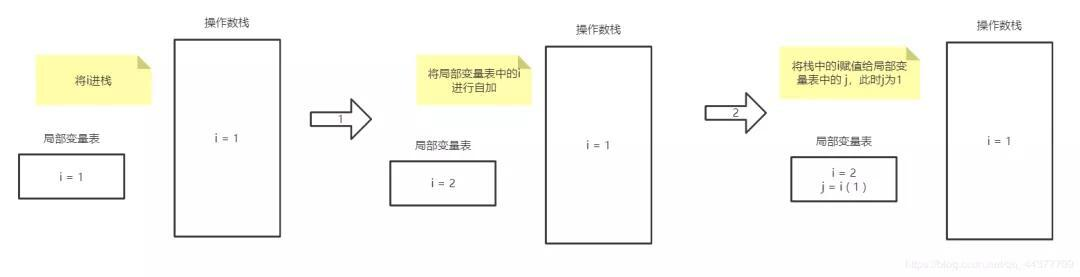# 2.4、第四步

int k = i + ++i * i++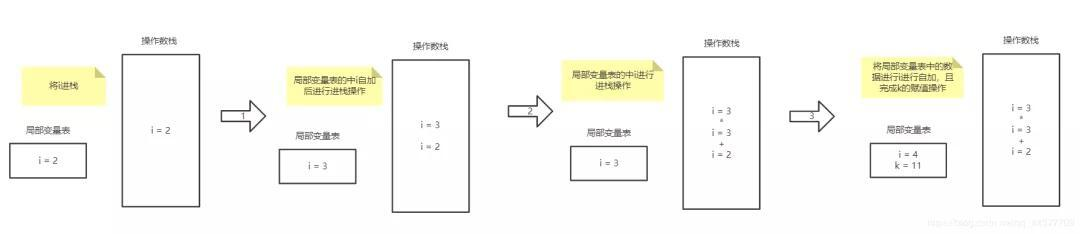# 2.5、结果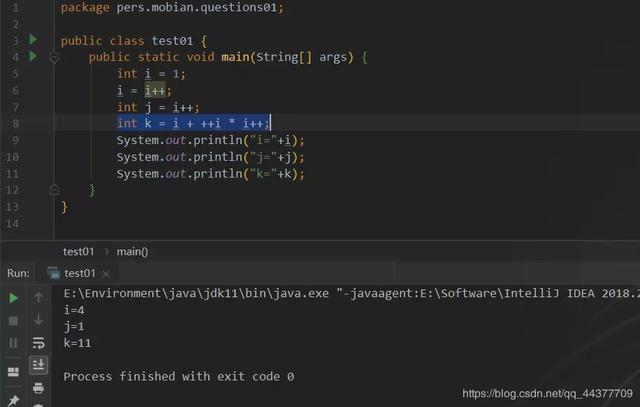# 3、i = ++i

public class test02 {    public static void main(String[] args) {        int i = 1;        i = ++i;        System.out.println(i); // 结果：i = 2    }}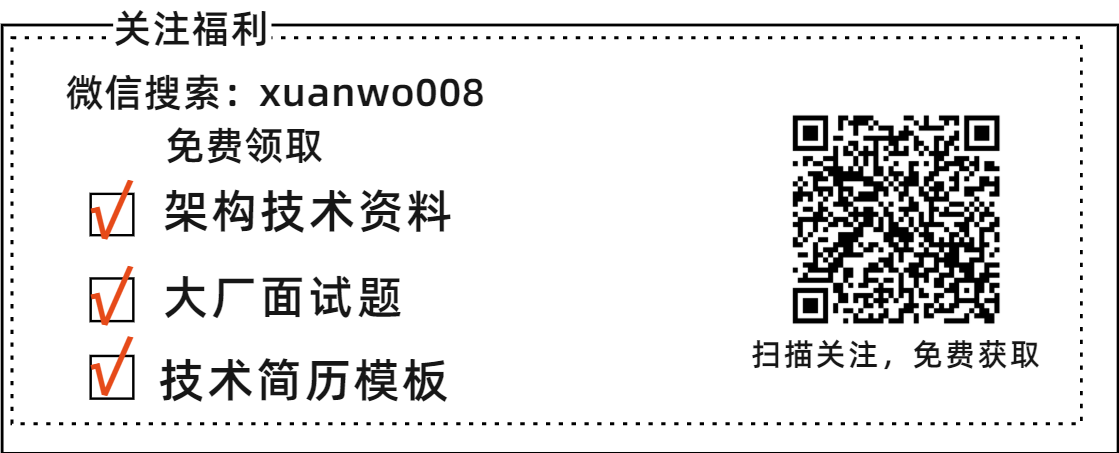# 需要资料的小伙伴，先转发，转发，转发，然后添加小助手VX：xuanwo008自取## 评论